# Learn to use Variables and Data Types in Python

1
4063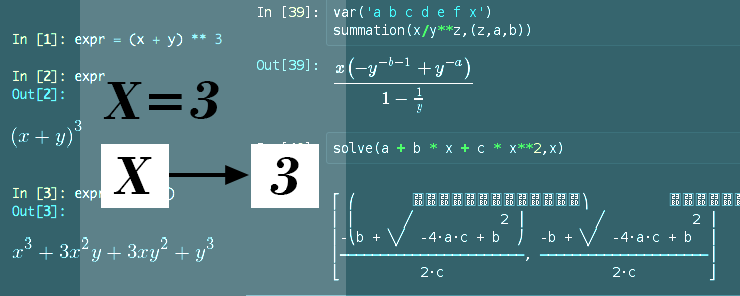In Python Tutorial Part (1), we had talked about the important features of the Python language that may encourage a developer to take his decision to use it. Then, we illustrated the Python installation procedure on both Windows and Linux. Finally, we ended the article with a simple one-line program that prints a simple text message.

In Python Tutorial Part (2), we did exactly what the article title says: we have concentrated on the hands-on to get the reader more familiar with Python, while showing some of the basic language syntax.

Now, we will talk about very important topic: Variables and Data Types. It is an important and vital one indeed, not only for Python developers, but also for any developer using any programming language.

Variables

Variables can be defined as named memory locations, which are used in computer programs to store values. As the name implies, the value stored in the memory location referenced by the variable name is changeable.

When a variable is declared, a memory segment at a specific address is allocated to that variable. The newly-allocated memory segment is marked as reserved until the variable is no longer needed. At that point, the memory segment is de-allocated and marked as Free.

A typical lifecycle of a variable could be:

1. The Variable is declared.
2. The variable is assigned an initial value.
3. The value stored in the variable is read and used in some data processing or decision making.
4. The variable is then assigned a new value.
5. The variable is read for processing or printing.
6. The variable is no longer needed, so the interpreter de-allocates the portion of memory it was occupying. This process is usually referred to as “Garbage Collection”.

Why Variables?

– What is the need for variables? And when do I have to use them?

Consider the following algebraic function:

f(x,y) = x + y

f is a function in two variables x and y. (i.e. its value depends on the value of both x and y). We need to substitute the values of x and y to get the resultant value of the function.

– All that headache only to add two numbers?! That is ridiculously easy!! Who may care about using variables for adding a couple of numbers?! It is something to do simply with eyes!!

Just wait and you will get the whole idea within few minutes. We need to repeatedly prompt the user for values of x and y, then the program will return the result, this will continue until the user enters 0 as the value for both x and y.

– Again, it is an addition, not a big deal!!

Okay, consider having to add two huge numbers like 5794256 and 856123. It is an addition!! Could you do it only with eyes?!

Now, consider the function to substitute the values in is something like this:

f(x,y,z) = 2×4 + 3xy3 + yz2 + 38

Got the idea?! All right.

* * * * * *

Identifiers

To use a variable, you have to know its name; so, it must be given a name. In the mathematical examples above: x, y, and z are variables’ names. As the variable names are used to identify different variables, they are considered identifiers. Valid variable names in Python should:

• Be one or more characters length, with the first character either an Upper/Lower case letter or an underscore.
• Have the other characters (other than the first) can be any alphanumeric characters, or underscore.
• Python is case-sensitive; i.e. counter, Counter, and COUNTER can be valid names for completely different variables within the same program.
• Not be a reserved word. The list of Python reserved words are:

if                      elif                   else                  and                  or                     del
from                None               return               try                    as                     global
pass                 True                 assert               not                   in                     nonlocal
while               break               except              import              with                 class
False                yield                continue          finally              is                      raise
def                   for                   lambda

As a best practice, it is advised to select meaningful variable names that indicate the purpose of the variable and what it is used for. That makes the code more readable. For example, variable names like word_count, grand_total, bench_number, firstname, and employee_id are considered meaningful. You could easily realize that the variable named firstname is a variable of the type String, which stores the first name of somebody.

Data Types

Each variable has a value stored in, which could be a number or a string of text. The “real” numbers themselves are divided into two main subtypes:

• Whole numbers (Integers).
• Floating-point numbers.

Numbers and Strings are the basic data types used in Python. So, let’s talk about them, and illustrate each type with examples.

Numbers

The following are two examples for variables holding numeric values:

x=4
y=2.5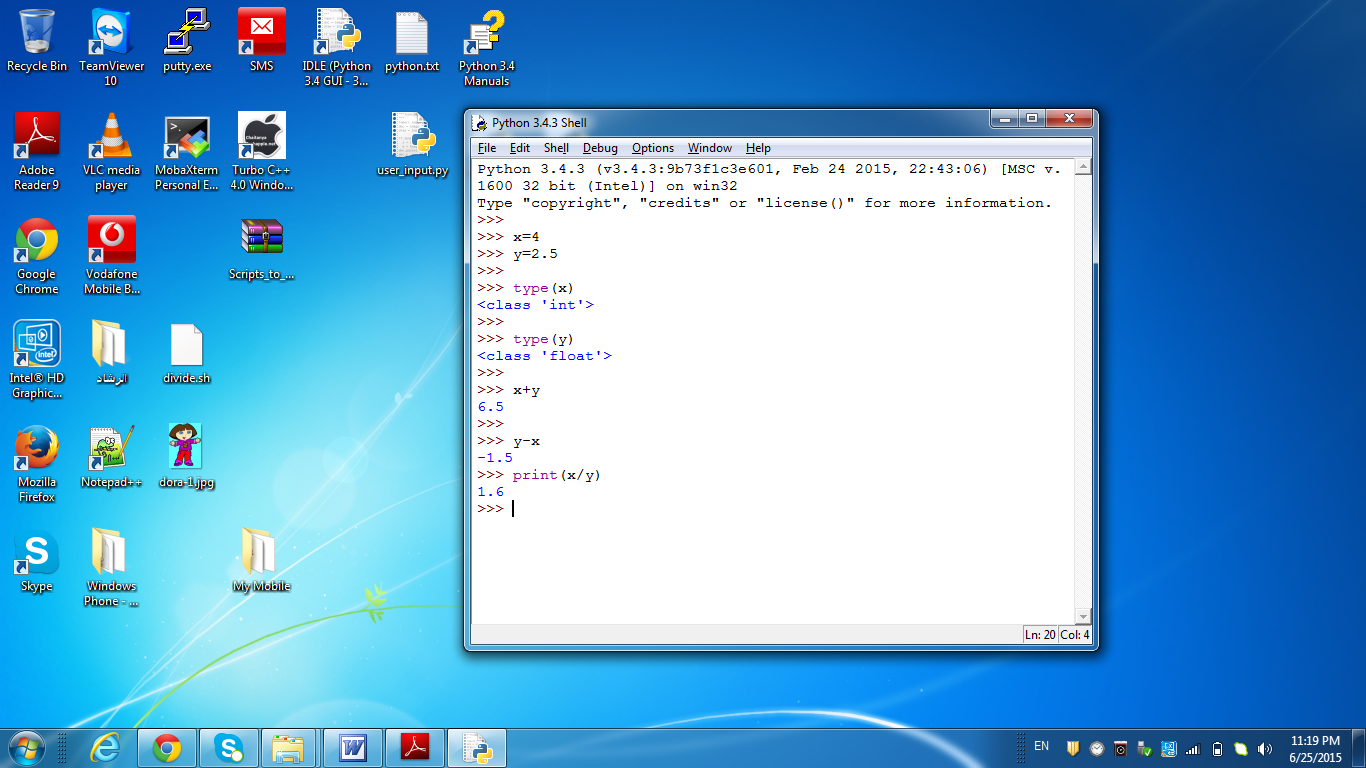Image 1: Numeric Variables

The statements x=4 and y=2.5 are called assignment statements. The assignment statement simply stores the value on the right side of the equal sign (called assignment operator) in the memory location represented by the variable name on the left side.

The Python function type (var) returns the data type of the argument passed to it. As you can see in the illustration above, the result of the statement type(x) shows that the variable x is of the type “int” (short for integer). Similarly, type(y) shows that y is a floating-point variable.

The arithmetic operations that are applicable to the numeric values are also applicable for use with variables of type’s int and float. So, a statement like x+y has exactly the same effect of the statement 4+2.5 that gives a result of 6.5

Note: When printing the value of a variable, make sure not to use quotes with it; i.e. if the variable is surrounded by single or double quotes, the print function deals with it literally by printing the name of the variable instead of its value.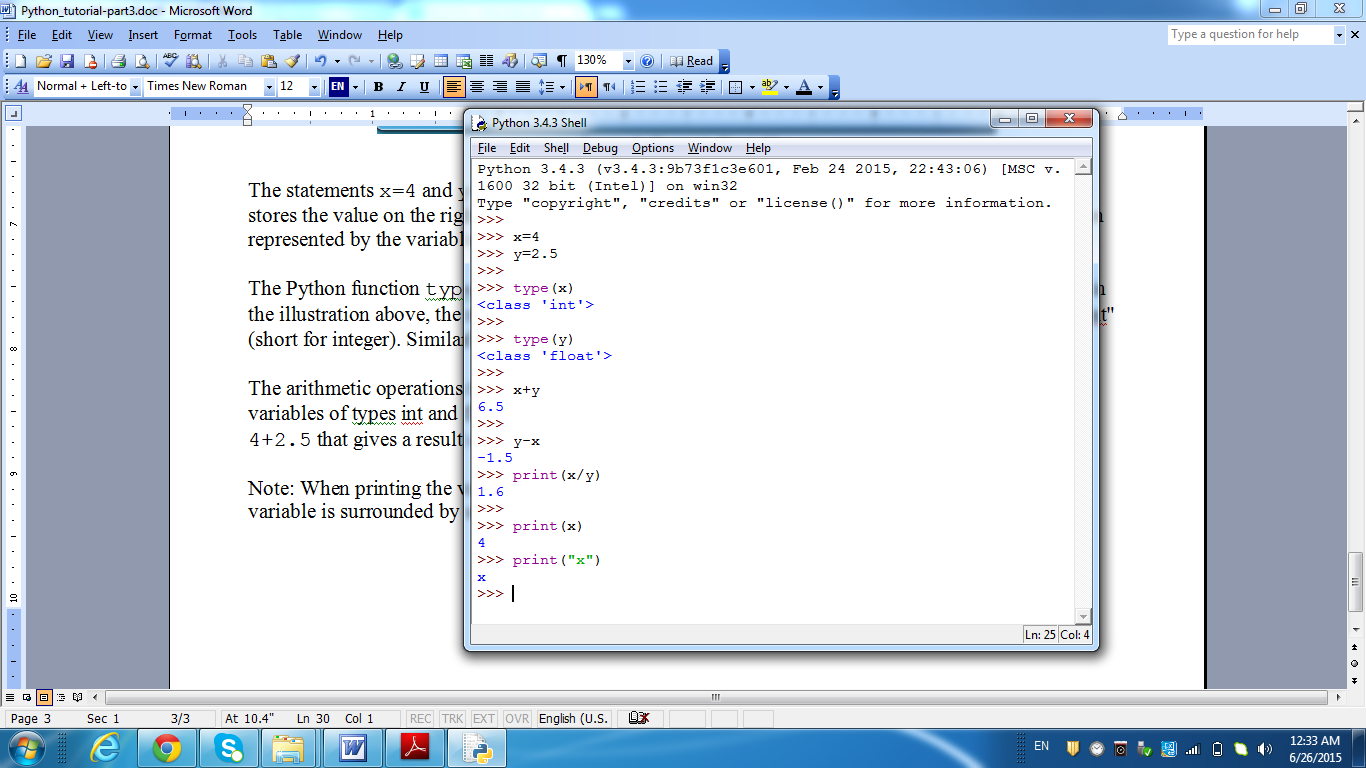Image 2:  Using quotes when printing variables causes the print function

to print the literal name of the variable (not its value)

Strings

A String is simply an array (sequence) of characters. Strings can be used to represent text information like: names, addresses, types, and descriptions.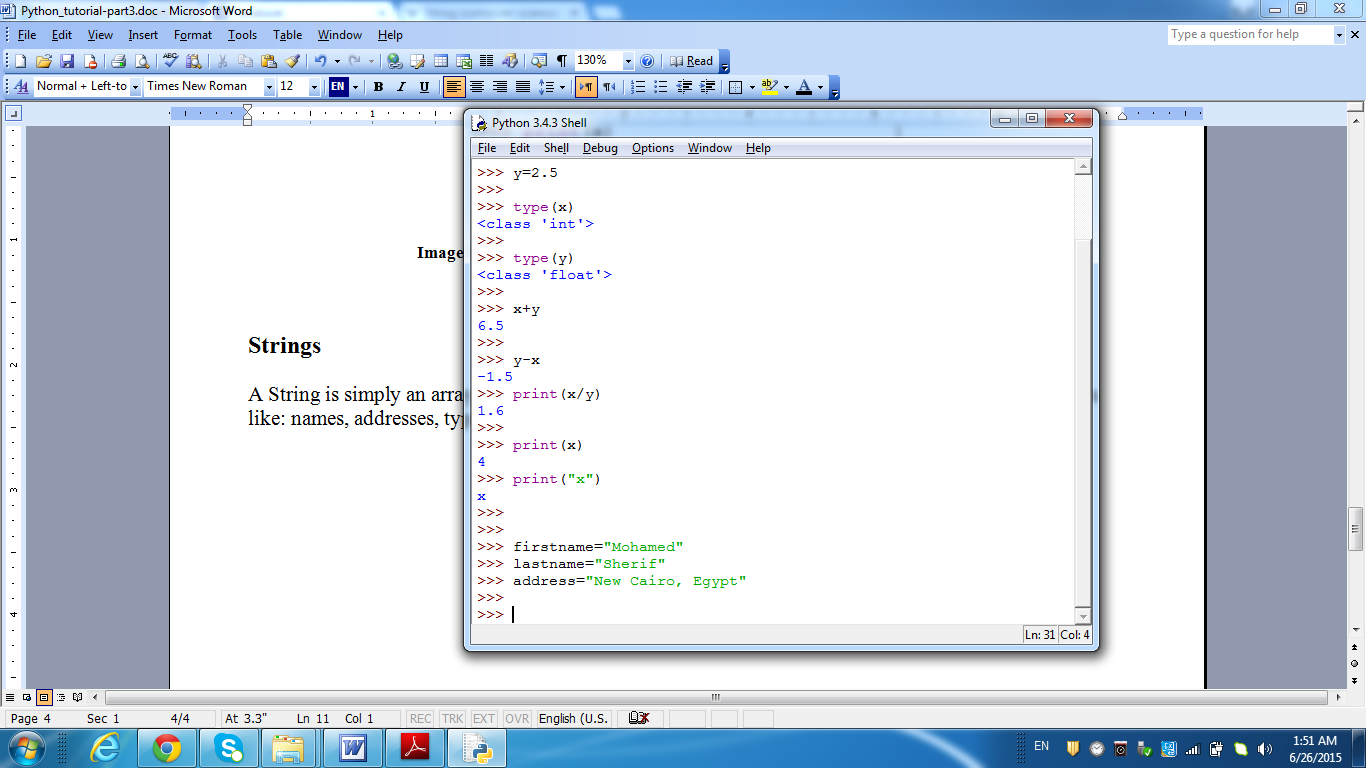Image 3: String Variables’ assignment.

Strings must be delimited using either single or double quotes. Like the numeric data types, strings also have operators and functions that handle most of the text processing needs. For example, the plus (+) operator when used with strings achieves strings concatenation: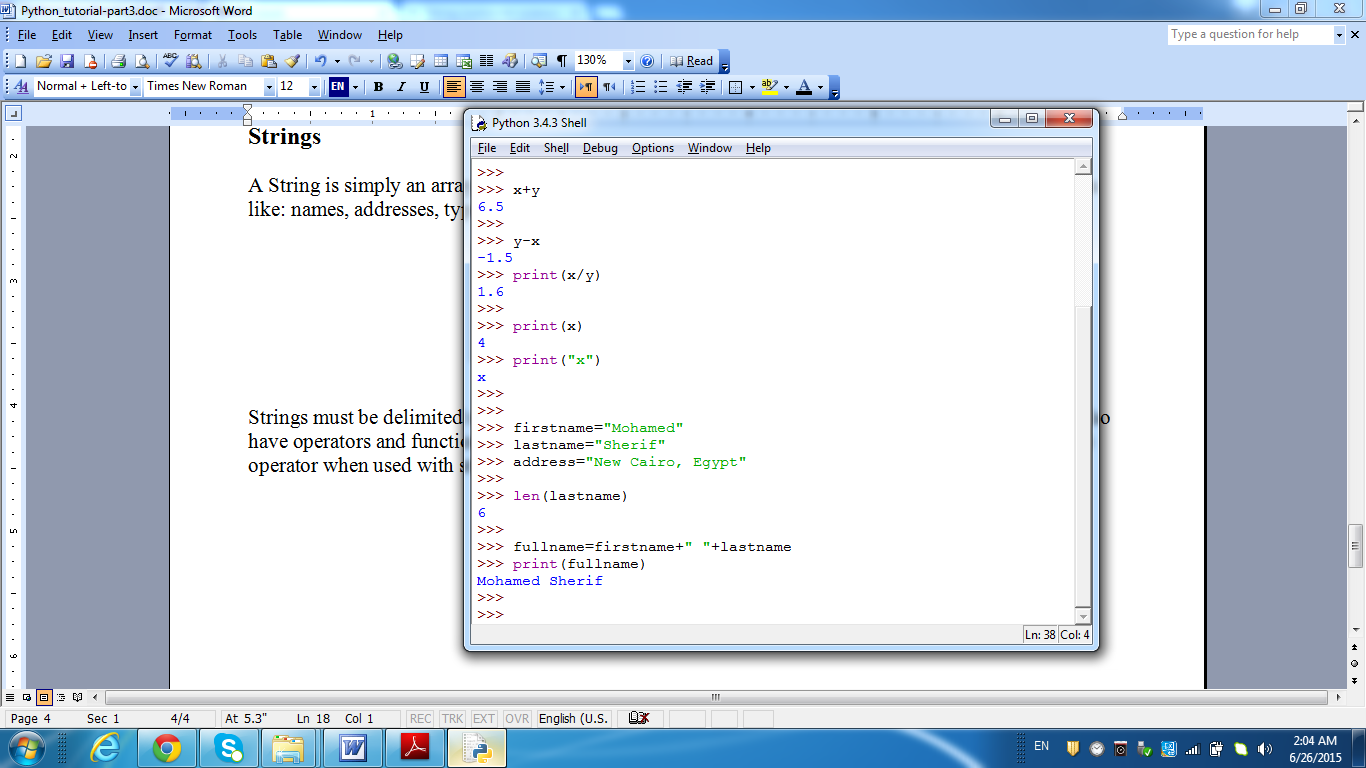Image 4: String Concatenation using the + operator

To get the length of a string, use the len(str) function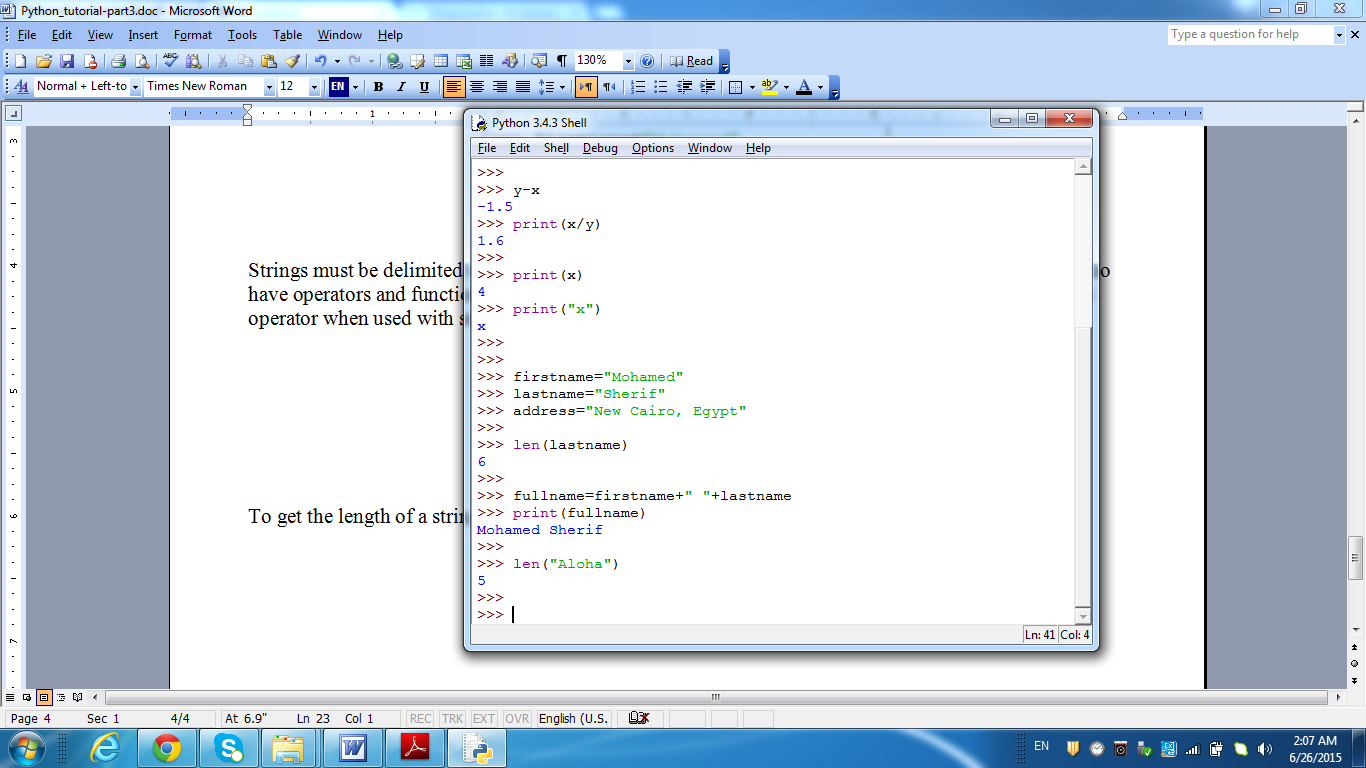Image 5: Using the len function to get the length of a string.

* * * * * *

In this article, we have talked about Variables, and the basic data types in Python. The concepts discussed in this article are very important for any developer in general, not Python developers only. In the next article, we will talk about another important topic “Arithmetic and Logical Operators“.

So, don’t miss it!!

#### 1 COMMENT

1. […] these features with some examples in an attempt to get you more familiar with Python. In the Python Tutorial Part 3, we will talk about variables in Python and the Data types. So, don’t go anywhere. We […]